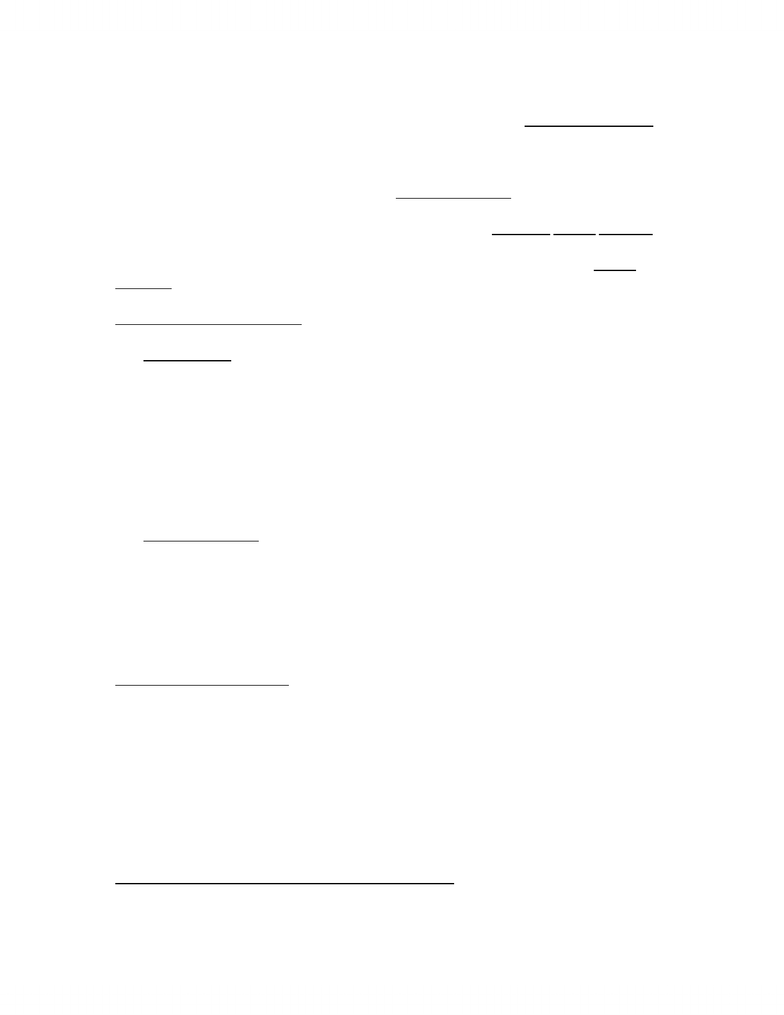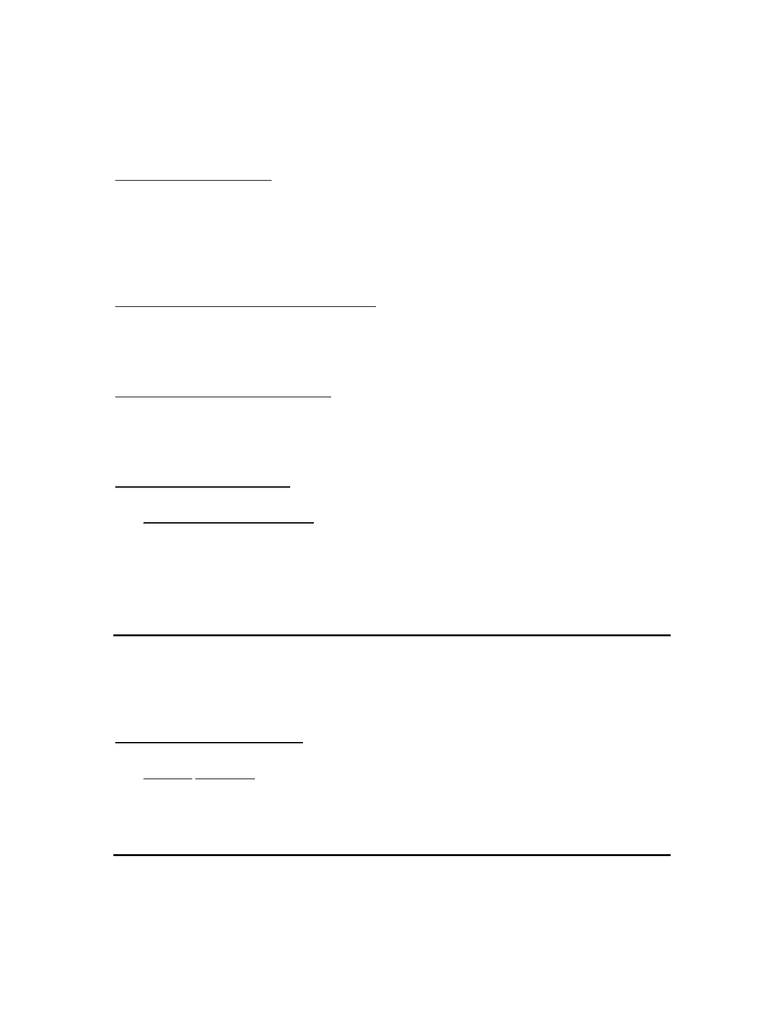# Chapters These notes cover all of chapter 4 and five in detail. Key terms, and concepts incorporated in an easy to follow format. Recommended for studying a midterm.

58 views10 pages
School
Department
CourseEcon Mid Term Review Chapter 4 and 5
Chapter 4 Elasticity
Chapter 5 Efficiency and Equity
Chapter 4 Review
The price elasticity of demand is a units-free measure of the responsiveness of the
quantity demanded of a good to a change in its price when all other influences on
Calculating Elasticity: The price elasticity of demand is calculated by using the
formula:
Percentage change in quantity demanded
------------------------------------------------------
Percentage change in price
To calculate the price elasticity of demand:
We express the change in price as a percentage of the average pricethe average of
the initial and new price, and we express the change in the quantity demanded as a
percentage of the average quantity demandedthe average of the initial and new
quantity.
By using the average price and average quantity, we get the same elasticity value
regardless of whether the price rises or falls. The ratio of two proportionate
changes is the same as the ratio of two percentage changes. The measure is units
free because it is a ratio of two percentage changes and the percentages cancel out.
Changing the units of measurement of price or quantity leave the elasticity value the
same.
The formula yields a negative value, because price and quantity move in opposite
directions. But it is the magnitude, or absolute value, of the measure that reveals
how responsive the quantity change has been to a price change.
Inelastic and Elastic Demand
Demand can be inelastic, unit elastic, or elastic, and can range from zero to infinity.
If the quantity demanded doesn’t change when the price changes, the price elasticity
of demand is zero and the good as a perfectly inelastic demand.
Unlock document

This preview shows pages 1-3 of the document.
Unlock all 10 pages and 3 million more documents.If the percentage change in the quantity demanded equals the percentage change in
price, the price elasticity of demand equals 1 and the good has unit elastic demand.
Elasticity values that have a percentage change in the quantity demanded that is
smaller than the percentage change in price so that the price elasticity of demand is
less than 1 and the good has what is called inelastic demand. IF the percentage
change in the quantity demanded is infinitely large when the price barely changes,
the price elasticity of demand is infinite and the good has perfectly elastic demand. If
the percentage change in quantity demanded is greater than the percentage change
in price, the price elasticity of demand is greater than 1 and the good has elastic
demand.
Total Revenue and Elasticity
The total revenue from the sale of good or service equals the price of the good
multiplied by the quantity sold. When the price changes, total revenue also changes
but a rise in price doesn’t always increase total revenue.
The change in total revenue due to a change in price depends on the elasticity of
demand: If demand is elastic, a 1 percent price cut increases the quantity sold by
more than 1 percent, and total revenues decreases. If demand is unit elastic, a 1
percent price cut increases the quantity sold by 1 percent, and total revenue
remains unchanged.
The total revenue test is a method of estimating the price elasticity of demand by
observing the change in total revenue that results from a price change (when all
other influences on the quantity sold remain the same).
If a price cut increases total revenue, demand is elastic.
If a price cut decreases total revenue, demand is inelastic.
If a price cut leaves total revenue unchanged, demand is unit elastic.
Expenditure and Elasticity
If your demand is elastic, a 1 percent price cut increases the quantity you buy
by more than 1 percent and your expenditure on the item increases.
If your demand is inelastic, a 1 percent price cut increases the quantity you
buy by less than 1 percent and your expenditure on the item decreases.
If your demand is unit elastic, a 1 percent price cut increases the quantity you
buy by 1 percent and your expenditure on the item does not change.
The Factors That Influence the Elasticity of Demand
The elasticity of demand for a good depends on:
Unlock document

This preview shows pages 1-3 of the document.
Unlock all 10 pages and 3 million more documents.The closeness of substitutes
The proportion of income spent on the good
The time elapsed since a price change
Closeness of substitutes
The closer the substitutes for a good or service, the more elastic are the demand for
it. Necessities, such as food or housing, generally having inelastic demand.
Luxuries, such as exotic vacations, generally have elastic demand.
Proportion of income spent on the good
The greater the proportion of income consumer’s spent on a good, the larger is its
elasticity of demand.
Time Elapsed Since Price Change
The more time consumers have to adjust to a price change, or the longer that a good
can be stored without losing its value, the more elastic is the demand for that good.
Cross Elasticity of Demand
The cross elasticity of demand is a measure of the responsiveness of a demand for a
good to a change in the price of a substitute or a compliment, other things remaining
the same.
The formula for calculating the cross elasticity is:
Percentage change in quantity demanded
Percentage change in price of substitute or compliment
The cross elasticity of demand for a substitute is positive.
The cross elasticity of demand for a complement is negative.
Income Elasticity of Demand
The income elasticity of demand measures how the quantity demanded of a good
responds to a change in income, other things equal. The formula for calculating the
income elasticity of demand is:
Percentage change in quantity demanded
Percentage change in income
Unlock document

This preview shows pages 1-3 of the document.
Unlock all 10 pages and 3 million more documents.